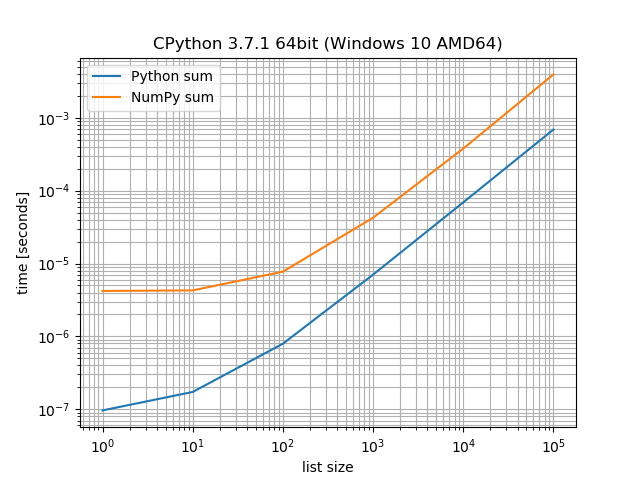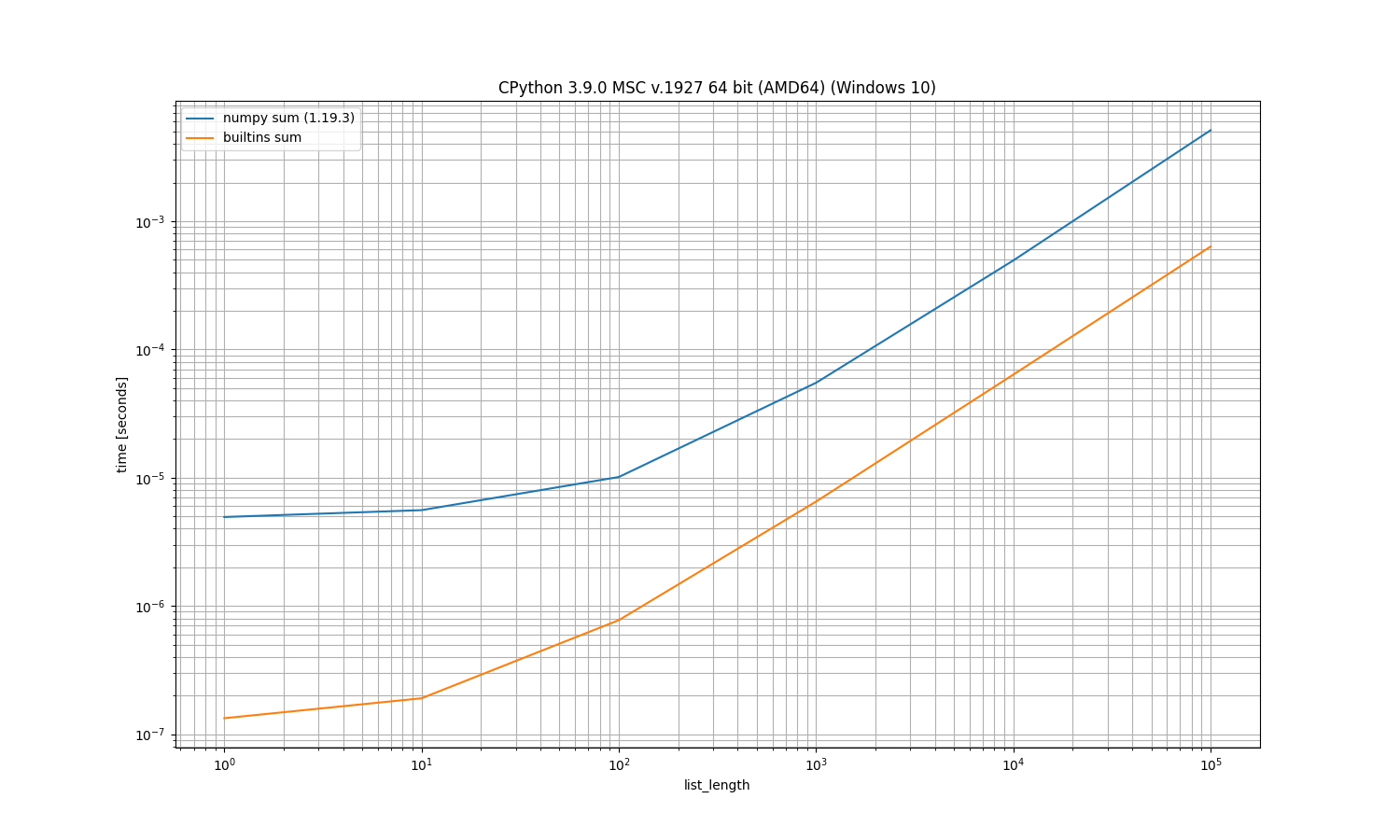# Welcome to simple_benchmark’s documentation!¶

## Installation¶

Using `pip`:

```python -m pip install simple_benchmark
```

Or installing the most recent version directly from `git`:

```python -m pip install git+https://github.com/MSeifert04/simple_benchmark.git
```

To utilize the all features of the library (for example visualization) you need to install the optional dependencies:

Or install them automatically using:

```python -m pip install simple_benchmark[optional]
```

## Getting started¶

Suppose you want to compare how NumPys sum and Pythons sum perform on lists of different sizes:

```>>> from simple_benchmark import benchmark
>>> import numpy as np
>>> funcs = [sum, np.sum]
>>> arguments = {i: *i for i in [1, 10, 100, 1000, 10000, 100000]}
>>> argument_name = 'list size'
>>> aliases = {sum: 'Python sum', np.sum: 'NumPy sum'}
>>> b = benchmark(funcs, arguments, argument_name, function_aliases=aliases)
```

The result can be visualized with `pandas` (needs to be installed):

```>>> b
Python sum  NumPy sum
1       9.640884e-08   0.000004
10      1.726930e-07   0.000004
100     7.935484e-07   0.000008
1000    7.040000e-06   0.000042
10000   6.910000e-05   0.000378
100000  6.899000e-04   0.003941
```

Or with `matplotlib` (has to be installed too):

```>>> b.plot()

>>> # To save the plotted benchmark as PNG file.
>>> import matplotlib.pyplot as plt
>>> plt.savefig('sum_example.png')
```## Command-Line interface¶

Warning

The command line interface is highly experimental. It’s very likely to change its API.

It’s an experiment to run it as command-line tool, especially useful if you want to run it on multiple files and don’t want the boilerplate.

File `sum.py`:

```import numpy as np

def bench_sum(l, func=sum):
return func(l)

def bench_numpy_sum(l, func=np.sum):
return np.sum(l)

def args_list_length():
for i in [1, 10, 100, 1000, 10000, 100000]:
yield i, *i
```

Then run:

```\$ python -m simple_benchmark sum.py sum.png
```

With a similar result `sum.png`: